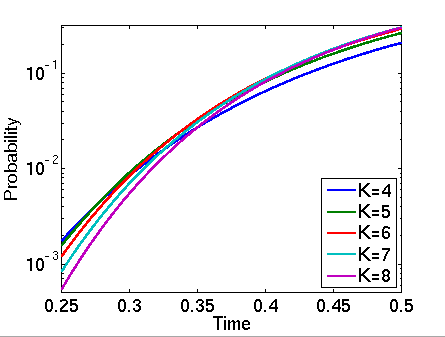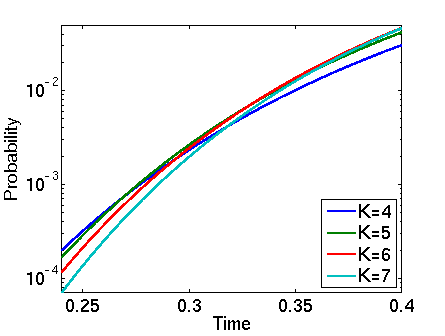# Simple Peer-To-Peer Protocol

### Introduction

This case study describes a simple peer-to-peer protocol based on BitTorrent. The model comprises a set of clients trying to download a file that has been partitioned into K blocks. Initially, there is one client that has already obtained all of the blocks and N additional clients with no blocks. Each client can download a block from any of the others but they can only attempt four concurrent downloads for each block.

Our model is represented as a CTMC. The PRISM source code for this case study (when N=4 and K=5) is given below.

```// Simple peer-to-peer file distribution protocol based on BitTorrent
// gxn/dxp Jan 2006

ctmc

// Actually there are N+1=5 clients, one of which has all blocks initially

const double mu=2;

// A client can download from the single client which starts with all blocks
formula rate1=mu*(1+min(3,b11+b21+b31+b41));
formula rate2=mu*(1+min(3,b12+b22+b32+b42));
formula rate3=mu*(1+min(3,b13+b23+b33+b43));
formula rate4=mu*(1+min(3,b14+b24+b34+b44));
formula rate5=mu*(1+min(3,b15+b25+b35+b45));

// client 1
module client1

b11 : [0..1];
b12 : [0..1];
b13 : [0..1];
b14 : [0..1];
b15 : [0..1];

[] b11=0 -> rate1 : (b11'=1);
[] b12=0 -> rate2 : (b12'=1);
[] b13=0 -> rate3 : (b13'=1);
[] b14=0 -> rate4 : (b14'=1);
[] b15=0 -> rate5 : (b15'=1);

endmodule

// construct remaining clients through renaming
module client2=client1[b11=b21,b12=b22,b13=b23,b14=b24,b15=b25,b21=b11,b22=b12,b23=b13,b24=b14,b25=b15] endmodule
module client3=client1[b11=b31,b12=b32,b13=b33,b14=b34,b15=b35,b31=b11,b32=b12,b33=b13,b34=b14,b35=b15] endmodule
module client4=client1[b11=b41,b12=b42,b13=b43,b14=b44,b15=b45,b41=b11,b42=b12,b43=b13,b44=b14,b45=b15] endmodule

// labels
label "done1" = b11+b12+b13+b14+b15 = 5; // client 1 has received all blocks
label "done2" = b21+b22+b23+b24+b25 = 5; // client 2 has received all blocks
label "done3" = b31+b32+b33+b34+b35 = 5; // client 3 has received all blocks
label "done4" = b41+b42+b43+b44+b45 = 5; // client 4 has received all blocks
label "done" = (b11+b12+b13+b14+b15)+(b21+b22+b23+b24+b25)+(b31+b32+b33+b34+b35)+(b41+b42+b43+b44+b45) = 20; // all clients have received all blocks

// reward: fraction of blocks received
rewards "frac_rec"
true : ((b11+b12+b13+b14+b15)/5)/4;
true : ((b21+b22+b23+b24+b25)/5)/4;
true : ((b31+b32+b33+b34+b35)/5)/4;
true : ((b41+b42+b43+b44+b45)/5)/4;
endrewards
```

### Model Statistics

The table below gives information about the CTMC representing the model: the number of states and the number of transitions.

 N: K: Model: States: Transitions: 4 4 65,536 524,288 5 1,048,576 10,485,760 6 16,777,216 201,326,592 7 268,435,456 3,758,096,384 8 4,294,967,296 68,719,476,736 5 4 1,048,576 10,485,760 5 33,554,432 419,430,400 6 1,073,741,824 16,106,127,360 7 34,359,738,368 601,295,421,440

### Model Construction Times

The table below gives the times taken to construct the models. This process involves first building a CTMC (represented as an MTBDD) from the system description (in our module based language), and then computing the reachable states using a BDD based fixpoint algorithm. The total time required and the number of fixpoint iterations are given below.

 N: K: Model: Time (s): Iter.s: 4 4 17 0.00 5 21 0.01 6 25 0.01 7 29 0.02 8 33 0.02 5 4 21 0.00 5 26 0.01 6 31 0.02 7 36 0.03

### Model Checking

We have model checked the following property:

• "the probability that all clients have received all blocks by time T"

The corresponding CSL formula is given by:

```const double T; // time bound

// probability that all clients have received all blocks by time T
P=? [ true U<=T  "done"  ]

// expected fraction of blocks received by time T
R=? [ I=T ]

```
• N=4 (full time range)• N=4 (zoom)• N=5 (full time range)• N=5 (zoom)